#### Equilibrium points and Lyapunov stability

At the very least, it seems that the state should remain fixed at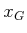, if it is reached. A point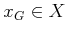is called an equilibrium point (or fixed point) of the vector field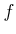if and only if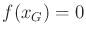. This does not, however, characterize how trajectories behave in the vicinity of. Let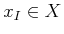denote some initial state, and let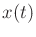refer to the state obtained at time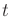after integrating the vector fieldfrom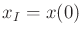.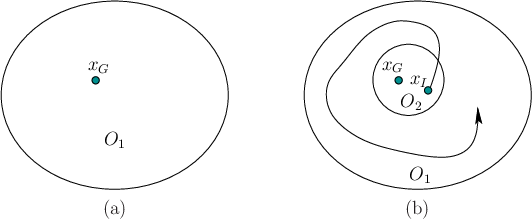See Figure 15.1. An equilibrium pointis called Lyapunov stable if for any open neighborhood15.1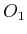ofthere exists another open neighborhood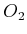ofsuch that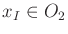implies that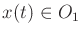for all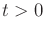. If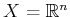, then some intuition can be obtained by using an equivalent definition that is expressed in terms of the Euclidean metric. An equilibrium point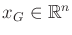is called Lyapunov stable if, for any, there exists some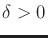such that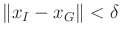implies that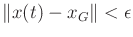. This means that we can choose a ball aroundwith a radius as small as desired, and all future states will be trapped within this ball, as long as they start within a potentially smaller ball of radius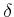. If a singlecan be chosen independently of every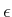and, then the equilibrium point is called uniform Lyapunov stable.

Steven M LaValle 2020-08-14